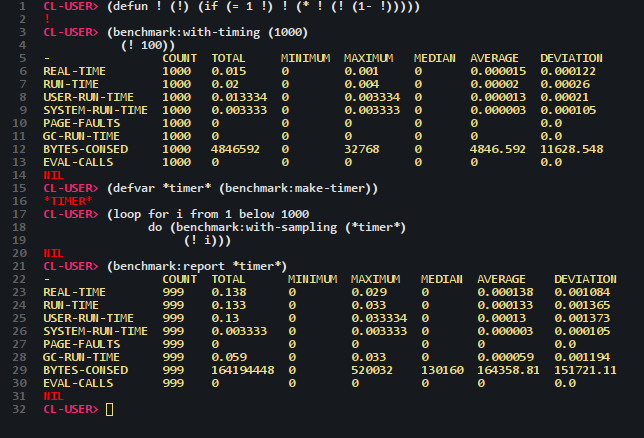# trivial-benchmark

``` git clone 'https://github.com/Shinmera/trivial-benchmark.git' (ql:quickload :trivial-benchmark) ```
4

Frequently I want to do a quick benchmark comparison of my functions. `TIME` is nice to get some data, but it's limited to a single run so there isn't really much of a statistical value in it. Trivial-benchmark runs a block of code many times and outputs some statistical data for it. On SBCL this includes the data from `TIME`, for all other implementations just the REAL- and RUN-TIME data. However, you can extend the system by adding your own `metric`s to it, or even by adding additional statistical `compute`ations.

## How To

For basic throwaway benchmarking, the `with-timing` macro should suffice:

``````(benchmark:with-timing (1000)
(+ 1 1))
``````

However, you can also do more complex timing using `make-timer` and `with-sampling`. The former creates a new timer object (with an optional list of metrics to sample) and the latter collects one sample for each metric of the timer for the duration of the body forms.

``````(defvar *timer* (benchmark:make-timer))

(benchmark:with-sampling (*timer*)
(+ 1 1))

(benchmark:with-sampling (*timer*)
(expt 10 100))

(benchmark:report *timer*)

(benchmark:reset *timer*)

(benchmark:report *timer*)
``````

Sample Output:## Extending Trivial-Benchmark

If you're interested in adding additional metrics, you'll want to take a look at the `metric` class, as well as the related methods, `start` `discard` `commit` `take-sample` `samples` `sample-size` `condense` `reduce-samples` `compute` `report` `reset`. For a basic `metric`, you only need to implement `start`, `discard`, `commit`, `samples`, and `take-sample`. The other functions have standard methods that will do their computations based on those five.

If you have a function that returns a current state of your sample and simply want to have the metric show the delta between `start` and `commit`, you can use `define-delta-metric`.

``````(define-delta-metric run-time
(/ (get-internal-run-time)
internal-time-units-per-second))
``````

You can also implement a new computation type that is used to display the different metrics in the table. For that, simply write a method on `compute` with the name of your computation as an eql-specializer and then push the name onto `*default-computations*` so that it's always displayed when using `report`. As an example, the maximum is simply calculated as follows:

``````(defmethod compute ((x (eql :maximum)) (metric metric))
(reduce-samples metric #'max))
``````